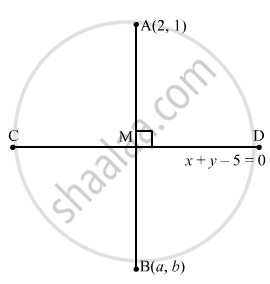Advertisement Remove all ads

# Find the Image of the Point (2, 1) with Respect to the Line Mirror X + Y − 5 = 0. - Mathematics

Answer in Brief

Find the image of the point (2, 1) with respect to the line mirror x + y − 5 = 0.

Advertisement Remove all ads

#### Solution

Let the image of A (2, 1) be B (a, b). Let M be the midpoint of AB.

$\therefore \text { Coordinates of M are } \equiv \left( \frac{2 + a}{2}, \frac{1 + b}{2} \right)$The point M lies on the line x + y − 5 = 0

$\therefore \frac{2 + a}{2} + \frac{1 + b}{2} - 5 = 0$

$\Rightarrow a + b = 7$         ... (1)
Now, the lines x + y − 5 = 0 and AB are perpendicular.
∴ Slope of AB $\times$ Slope of CD = −1

$\Rightarrow \frac{b - 1}{a - 2} \times \left( - 1 \right) = - 1$

$\Rightarrow a - 2 = b - 1$

⇒ $a - b = 1$  ... (2)
Adding eq (1) and eq (2):

$2a = 8$

$\Rightarrow a = 4$

Now, from equation (1):

$4 + b = 7$

$\Rightarrow b = 3$

Hence, the image of the point (2, 1) with respect to the line mirror x + y − 5 = 0 is (4, 3).

Is there an error in this question or solution?
Advertisement Remove all ads

#### APPEARS IN

RD Sharma Class 11 Mathematics Textbook
Chapter 23 The straight lines
Exercise 23.12 | Q 12 | Page 93
Advertisement Remove all ads

#### Video TutorialsVIEW ALL 

Advertisement Remove all ads
Share
Notifications

View all notifications

Forgot password?